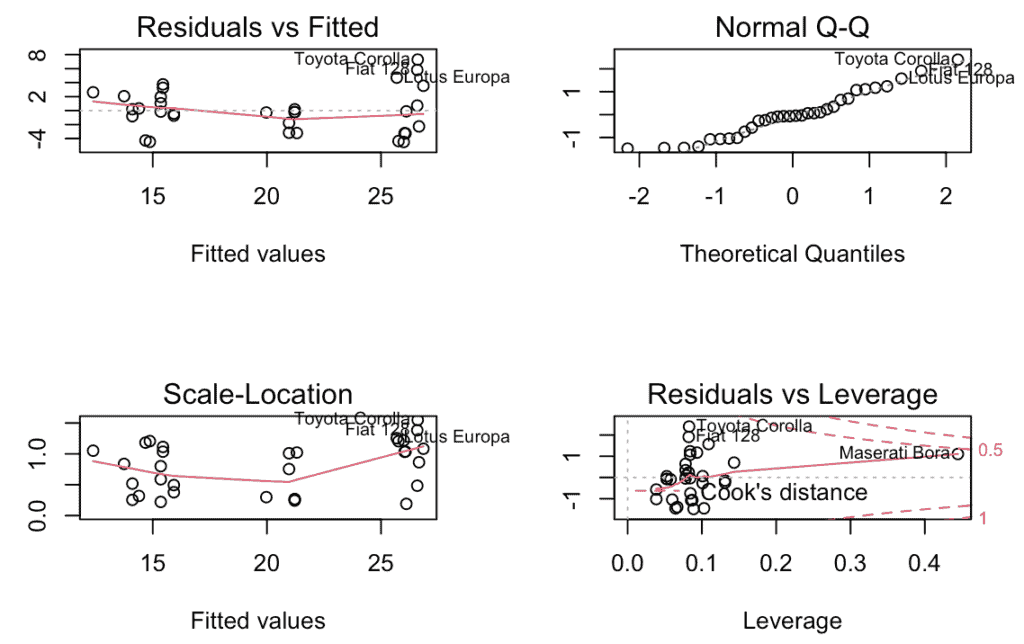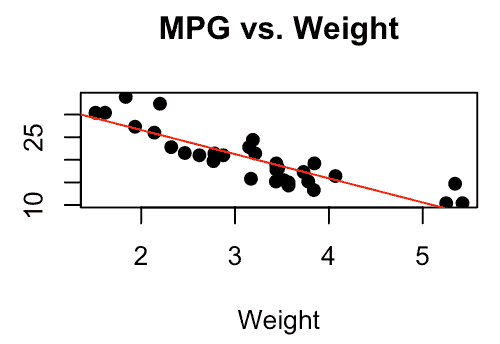# How to Use the lm() Function in R to Fit Linear Models

The lm() function in R is “used to create a linear regression model, which you can use for regression analysis”.

### Syntax

``lm(formula, data, ...)``

### Parameters

1. formula: The formula for the linear model (e.g. y ~ x1 + x2)
2. data: The name of the data frame that contains the data.
3. : Additional arguments, such as weights or contrasts.

## R program of lm() function

``````# Load the dataset
data(mtcars)

# Fit a linear model with miles per gallon (mpg) as the dependent
# Variable and weight (wt) and horsepower (hp) as independent variables
model <- lm(mpg ~ wt + hp, data = mtcars)

# Display the summary of the model
summary(model)
``````

Output

``````Call:
lm(formula = mpg ~ wt + hp, data = mtcars)

Residuals:
Min 1Q Median 3Q Max
-3.941 -1.600 -0.182 1.050 5.854

Coefficients:
Estimate Std. Error t value Pr(>|t|)
(Intercept) 37.22727 1.59879 23.285 < 2e-16 ***
wt -3.87783 0.63273 -6.129 1.12e-06 ***
hp -0.03177 0.00903 -3.519 0.00145 **
---
Signif. codes: 0 ‘***’ 0.001 ‘**’ 0.01 ‘*’ 0.05 ‘.’ 0.1 ‘ ’ 1

Residual standard error: 2.593 on 29 degrees of freedom
Multiple R-squared: 0.8268, Adjusted R-squared: 0.8148
F-statistic: 69.21 on 2 and 29 DF, p-value: 9.109e-12
``````

The “summary()” function provides information about the model fit, including coefficients, standard errors, t-values, and p-values.

## Use predict() function with confint() function

You can use the “predict()” function to make predictions based on the fitted model and other functions like “confint()” to compute confidence intervals for the model coefficients.

``````data(mtcars)

model <- lm(mpg ~ wt + hp, data = mtcars)

new_data <- data.frame(wt = c(2.5, 3.0), hp = c(100, 150))

predicted_mpg <- predict(model, newdata = new_data)

print(predicted_mpg)``````

Output

``````   1          2
24.35540   20.82784
``````

## View Diagnostic Plots of Model created by lm() function

Several diagnostic plots can be generated in R to examine the quality of a linear regression model created using the lm() function. You can use the plot() function to create these plots.

Run the below code in RStudio.

``````# creating a linear regression model
data(mtcars)
model <- lm(mpg ~ cyl + hp, data = mtcars)

# plotting diagnostic plots
par(mfrow = c(2, 2)) # setting the plotting area into a 2x2 grid
plot(model)``````

Output## Plot the Fitted Regression Model

Once you have created a linear regression model using the lm() function in R, you can plot the fitted regression line on top of your data.

``````# load the mtcars dataset
data(mtcars)

# create a linear regression model
model <- lm(mpg ~ wt, data = mtcars)

# create a scatterplot of the data
plot(mtcars\$wt, mtcars\$mpg, main="MPG vs. Weight",
xlab="Weight", ylab="Miles per Gallon", pch=19)

abline(model, col="red")
``````

Output## Use the Regression Model to Make Predictions

Once you have created a regression model using the lm() function in R, you can use the predict() function to make predictions with that model.

``````# load the mtcars dataset
data(mtcars)

# create a linear regression model
model <- lm(mpg ~ wt, data = mtcars)

# make a prediction
new_data <- data.frame(wt = c(2.5, 3.0, 3.5))
predictions <- predict(model, new_data)

print(predictions)``````

Output

``````   1          2        3
23.92395  21.25171  18.57948``````

That’s it.